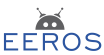#Real-Time Robotics Framework

### Site Tools

tools:matrix:start

# Vectors and Matrices

The blocks in the control system are connected among each other with the aid of signals. As described in Signals they carry a timestamp and a value. The value could be a simple type such as an integer or float. Quite often it will consist of a vector or a matrix. A three dimensional vector of type double can be declared and initialized as follows:

Vector<3, double> v;

The declaration could be simplified to

Vector3<> v;

as Vector3 is a predefined type with three dimensions and the default element type is double. A Vector3 is identical to the type Matrix<3,1,double>. The vector could be initialized as follows

v = {1.5, -2, 0}
v << 1.5, -2, 0; // or with input operator
Vector3 v{1,2,3}; // or directly upon declaration

A matrix of 3 times 3 with element type int could be defined as

Matrix<3, 3, int> m;
m << 1, 2, 3,
4, 5, 6,
7, 8, 9;

The first three numbers will be filled into the first row. While the internal representation is simply a one dimensional vector, the matrix could be visualized as

col0 col1 col2 1 2 3 4 5 6 7 8 9

When declaring and initializing a matrix, the values must be given colon per colon. The same matrix as given above would be initialized as follows

Matrix<3, 3, int> m{1, 4, 7, 2, 5, 8, 3, 6, 9};

## Accessing Elements

You can access rows, columns or single elements of matrices with the following methods:

m.get(0,0);  // returns element, 1
m.get(1,0);  // returns element, 4
m.getRow(1);  // returns row, [4,5,6]
m.getCol(0);  // returns column, [1,4,7]'
m.getSubMatrix<2,2>(0,1);   // returns matrix [[2,5][3,6]]

Single elements, rows or columns can be written with the methods set(), setRow(), or setCol().

The operators () and [] work as well as can be seen by the following example

m(3);   // returns element or sets element with index 3
m;   // returns element or sets element with index 3
m(2,1);   // returns element or sets element with index 7
m[2,1];   // does not work, as the [] operator cannot be defined for two parameters

## Logging Matrices

A matrix can be logged simply by writing

log.info() << m;

With the 3 x 3 matrix from above we would get

2021-09-16 17:36:53:929    I:  [ [1 4 7]' [2 5 8]' [3 6 9]' ]

The matrix is plotted colon per colon. To give some more examples:

Matrix<1,1> m1{1};
Matrix<2,1> m2{1,2};
Matrix<1,2> m3{1,2};

This matrices will be printed as

2021-09-16 17:37:18:122    I:  '
2021-09-16 17:37:18:122    I:  [1 2]'         // one colon with two rows
2021-09-16 17:37:18:122    I:  [ ' ' ]' // one row with two colons

## Matrix Operations

Some examples show basic matrix operations.

Vector2 v1{1,2};
Matrix<1,2> v2{3,4};
log.info() << v1 * v2;            // will print [ [3 6]' [4 8]' ]
log.info() << v2 * v1;            // will print '

Matrix<2,2,double> m1{1,1.5,-1,2};
log.info() << v2 * m1;            // will print [ ' ' ]
log.info() << v1.transpose() * m1;// will print [ ' ' ]

auto x = v2 * m1;
Vector2 v4 = x.transpose();
log.info() << v4;                 // will print [9 5]'